### Getting used to ladder programming by starting with Ex-or gate.

So if you are reading this you should probably already know why and where is Ladder programming used.
Ladder programming is the most common and easiest programming language in PLC. And almost anyone can learn it in a matter of time. You just have to be good with logic.

To start off we'll learn how to program Rockwell Automation's Allen Bradley PLC. We are going to use RSlogix 500 software for programming the PLC.

For the starters, we'll see how to implement any one of the logic gates to understand the programming language.

There are two inputs and a single output in an Exclusive-or Gate. The truth table of the ex-or gate looks something like this,

 Input 1 Input 2 Output 0 0 0 0 1 1 1 0 1 1 1 0

There are two contacts in general in a ladder programming language.

Normally open -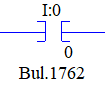Which looks something like the above image. So basically that depicts that initially, the address is in '0' or false state.

Normally Closed-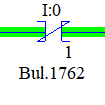So normally closed depicts that initially, the address is in '1' or true state.

Now that we know the basics we will jump to program the ex-or gate.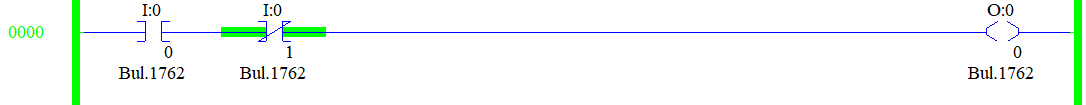This is how our first rung will look like. Let's see if it works logically.

I:0/0 is our first input and I:0/1 will be our second input.

O:0/0 is our output.

So the first condition of our table is both the inputs are 0 and the output is 0. Which is satisfied with our program.

The next condition will be the first input is 0 and the second input is 1 then the output should be true. But when we simulate it looks something like this,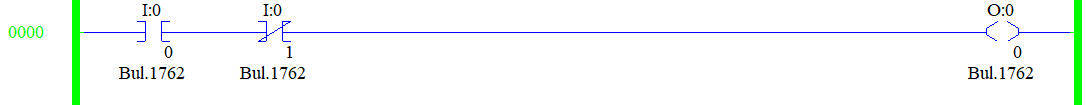Yes, so when I make my input 2 to go true the Normally closed address will become a Normally open address. Hence the output is zero. So our program is not satisfying the second conditioning.

Let's check whether our program matches the third condition which says the input 1 is made true and input 2 is false and the output should be true.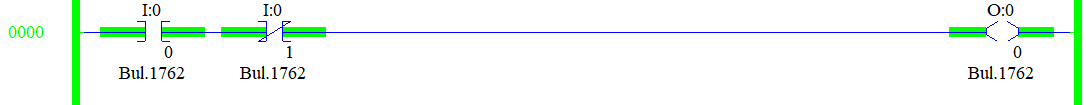Referring to the above rung you know that our program satisfies the third condition.

The last condition is we are going to make both the inputs true and the output should be false. Let's check if our program satisfies the last condition.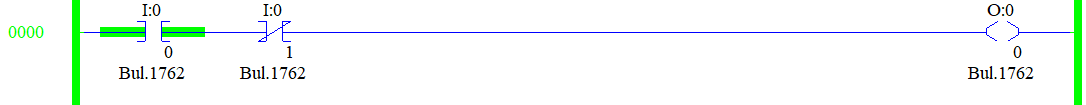Yes, our program satisfies the last condition also.

The only problem is our program is not satisfying the second condition let's fix this.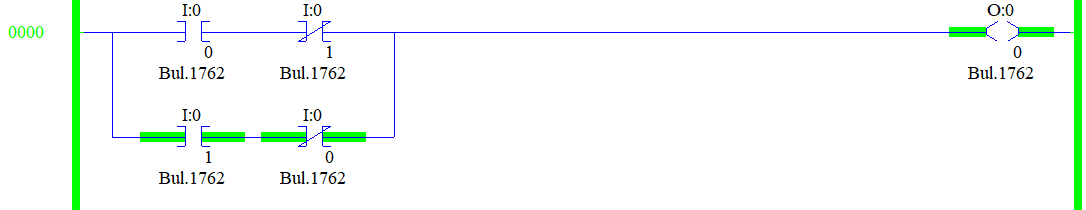This is our final program for the Ex-or gate and Refer to the above rung and let me know on the comments on what condition we used there to check our program?